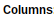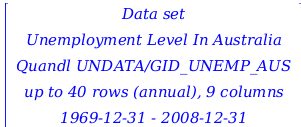Graph Theory - Maple Help

 GraphTheory

Maple 2019 enhances the GraphTheory package with new functions, including:

 •
 •
 •
 •
 •
 •

The SpecialGraphs subpackage also includes commands for eleven new graphs and many new options for customizing the visualizations of graphs have been added to the DrawGraph command.ExamplesFindVertexCover

FindVertexCover returns a list of vertices which comprise a vertex cover  in the graph G. The optional parameter size specifies a size for the clique.

 > $\mathrm{with}\left(\mathrm{GraphTheory}\right):$
 > $G≔\mathrm{CompleteGraph}\left(3,4\right)$
 ${G}{≔}{\mathrm{Graph 1: an undirected unweighted graph with 7 vertices and 12 edge\left(s\right)}}$ (1.1.1)
 > $\mathrm{DrawGraph}\left(G\right)$> $\mathrm{FindVertexCover}\left(G,3\right)$
 $\left[{1}{,}{2}{,}{3}\right]$ (1.1.2)
 > $\mathrm{FindVertexCover}\left(G,4\right)$
 $\left[{4}{,}{5}{,}{6}{,}{7}\right]$ (1.1.3)MaximumClique

MaximumClique returns a clique of the largest possible size in the specified graph. Maple 2019 includes a new heuristic, method=sat, which transforms the graph into an instance of the Boolean satisfiability problem which it then solves using the Logic[Satisfy] command.
The new default heuristic, method=hybrid, runs the two methods optimal and sat in parallel and returns the result of whichever method finishes first.

 > $M≔\mathrm{SpecialGraphs}:-\mathrm{MirzakhaniGraph}\left(\right)$
 ${M}{≔}{\mathrm{Graph 2: an undirected unweighted graph with 63 vertices and 183 edge\left(s\right)}}$ (1.2.1)
 > $\mathrm{MaximumClique}\left(M,\mathrm{method}=\mathrm{sat}\right)$
 $\left[{61}{,}{62}{,}{63}\right]$ (1.2.2)WattsStrogatzGraph

The WattsStrogatzGraph command permits the generation of random graphs with the Watts-Strogatz model, which produces graphs with a number of desirable real-world properties such as short average path lengths and high clustering.

 >
 ${\mathrm{WSG}}{≔}{\mathrm{Graph 3: an undirected unweighted graph with 20 vertices and 40 edge\left(s\right)}}$ (1.3.1)
 >New Special Graphs

The SpecialGraphs subpackage now includes built-in commands to generate the following special graphs or graph families:

 > $\mathrm{with}\left(\mathrm{SpecialGraphs}\right):$

Brinkmann Graph

Cameron Graph

Circulant Graph

Dürer Graph

 >>
 ${\mathrm{CG}}{≔}{\mathrm{Graph 4: an undirected unweighted graph with 231 vertices and 3465 edge\left(s\right)}}$ (2.1)
 > $\mathrm{NumberOfVertices}\left(\mathrm{CG}\right)$
 ${231}$ (2.2)
 > $\mathrm{NumberOfEdges}\left(\mathrm{CG}\right)$
 ${3465}$ (2.3)
 >>Friendship Graph

Hall-Janko Graph

Johnson Graph

Livingstone Graph

 >>
 ${\mathrm{HJG}}{≔}{\mathrm{Graph 5: an undirected unweighted graph with 100 vertices and 1800 edge\left(s\right)}}$ (2.4)
 > $\mathrm{NumberOfVertices}\left(\mathrm{HJG}\right);$
 ${100}$ (2.5)
 > $\mathrm{NumberOfEdges}\left(\mathrm{HJG}\right);$
 ${1800}$ (2.6)
 >>
 ${\mathrm{LG}}{≔}{\mathrm{Graph 6: an undirected unweighted graph with 266 vertices and 198 edge\left(s\right)}}$ (2.7)
 > $\mathrm{Girth}\left(\mathrm{LG}\right)$
 ${5}$ (2.8)

Suzuki Graph

Sylvester Graph

Tietze Graph



 >
 ${\mathrm{SG}}{≔}{\mathrm{Graph 7: an undirected unweighted graph with 1782 vertices and 370656 edge\left(s\right)}}$ (2.9)
 > $\mathrm{NumberOfEdges}\left(\mathrm{SG}\right)$
 ${370656}$ (2.10)
 >>New Visualization Options

The DrawGraph command and various graph highlighting commands have been enhanced with even more new ways to customize graph display. Some of the defaults have changed so that the vertex shape will generally be closer to the size of the vertex label, vertex borders will not overlap, and the size option passed to DrawGraph will be used to make graphs look better at sizes different than the default. As well, the arrows on directed edges are now draw with a more typical solid triangle shape with new stylesheet options to customize the arrow positions and size.

 >
 ${\mathrm{GV1}}{≔}{\mathrm{Graph 8: a directed weighted graph with 5 vertices and 5 arc\left(s\right)}}$ (3.1)
 >A new vertex shape option is now available as well, the shape can be any string understood by the new command plottools:-polygonbyname.

 >>There is a new option to control the amount of padding (measured in font points) around vertex labels.  This is useful if the automatically selected size of the vertex shape is too large or too small.

 >There is a new option to set the line style of graph edges.  Any linestyle from plot/options is supported.

 >In cases where the graph has many vertices, labels are turned off by default but plot annotations are added so that you can see the labels when you hover the mouse pointer over a vertex.

 >
 ${\mathrm{TV1}}{≔}{\mathrm{Graph 9: an undirected unweighted graph with 100 vertices and 99 edge\left(s\right)}}$(3.2)

The DrawGraph tree style can now be used on graphs that are not trees. This is implemented by choosing a vertex layout based on the SpanningTree starting at a specified root.

 >
 ${\mathrm{GV2}}{≔}{\mathrm{Graph 16: an undirected unweighted graph with 7 vertices and 10 edge\left(s\right)}}$